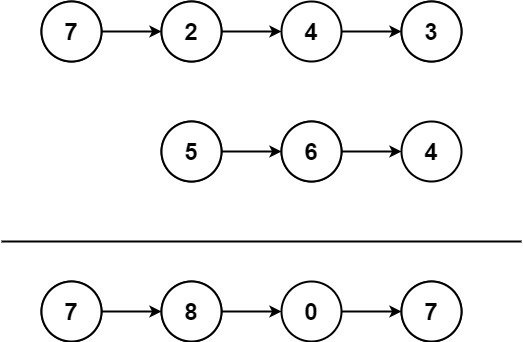# Leetcode 445 - Add two nums II

Note:

• Reversing linked lists are too naive.
• Use stacks to store vals and sum them up while popping them out.

Question:

You are given two non-empty linked lists representing two non-negative integers. The most significant digit comes first and each of their nodes contains a single digit. Add the two numbers and return the sum as a linked list.

You may assume the two numbers do not contain any leading zero, except the number 0 itself.

Example:Code: